# Line Plot - Definition with Examples

The Complete K-5 Math Learning Program Built for Your Child

• 40 Million Kids

Loved by kids and parent worldwide

• 50,000 Schools

Trusted by teachers across schools

• Comprehensive Curriculum

Aligned to Common Core

## Line Plot Games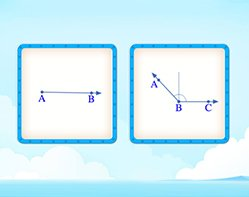Lines, Rays and Line Segments

Identify and distinguish between lines, rays and line segments. Did you know, that the sides of closed figures like rectangles are actually line segments.

Covers Common Core Curriculum 4.G.1

## What is a line plot?

A Line plot can be defined as a graph that displays data as points or check marks above a number line, showing the frequency of each value.

Here, for instance, the line plot shows the number of ribbons of each length.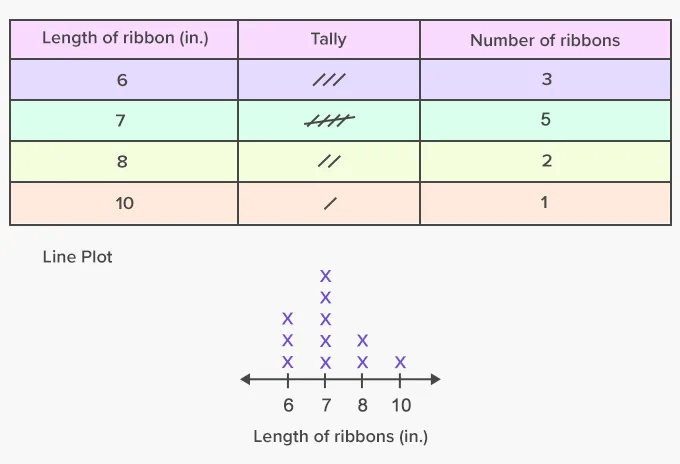Fun Facts A line plot is often confused with a line graph. A line plot is different from a line graph.

##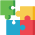Let's sing!

To draw a line plot,
A number line to ought,
The different values we sort.
Making data easy to spot!

##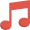Let's do it!

Ask your child to jot down the total number of money he spends daily during week. Ask him to represent the data using a line plot.

##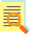Related math vocabulary

Won Numerous Awards & Honors